Optics Exam3 and Problem Solutions

Optics Exam3 and  Problem Solutions

1. Ray I comes to optical system as shown in the figure given below. Ray reflects from the mirror placed inside the sphere in 1 direction. How much we must rotate mirror to make reflected ray follows path 2.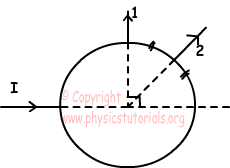Since reflected ray rotates 450, mirror must rotate 22,50

45/2=22,50

2. An observer is placed at point O and an obstacle is placed at point A. Which points shown in the figure below can be seen by observer?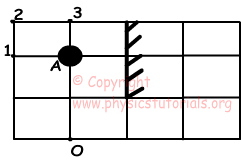Picture, given below, shows which points are in the viewpoint of observer. Observer can only see image of object 3.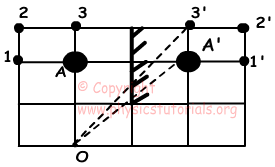3. Draw reflection path of the following ray from plane mirror and concave mirror.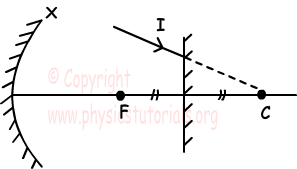Path of the ray is given below.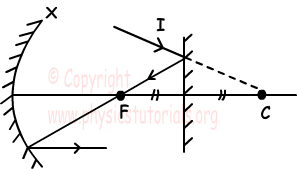4. An object is placed in front of a concave mirror. If the distance between object and its image is 48 cm, find the focal point of the mirror.(Himage=Hobject/3)

Since height of the image is smaller than height of the object, object must be placed away from the center of the mirror and image is formed between focal point and center of the mirror.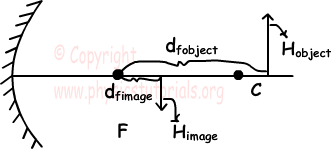Himage=Hobject/3

dfobject-dfimage=48cm

Hobject/Himage=dfobject/f=3

dfobject=3f

Hobject/Himage=f/dfimage=3

dfimage=f/3

dfobject-dfimage=48cm

3f-f/3=48cm

f=18cm

5. As you can see in the picture given below, ray comes to the first mirror, reflects and hits second mirror. If this ray turns back on itself after reflecting from the second mirror, find the focal point of the second mirror.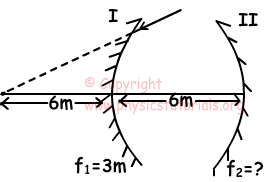Ray passes from the point A after reflecting from first mirror. We do our calculations by taking point A object of second mirror.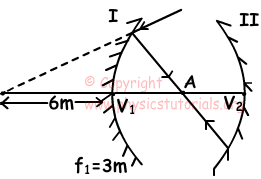1/f=1/dobject-1/6

1/3+1/6=1/dobject

dobject=2m

Point A is the center of the second mirror.

V1V2=6m

Center of the second mirror=4m=2f

f=2m

Author: